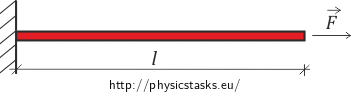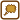## Wire Diameter Difference during its Stretching

Wire of an original length of 10 m is fixated at one end and is being stretched with force of 200 N which prolongs it by 4 mm. Find the original diameter of the wire and its difference during the stretching. Young’s modulus at strain of the wire is 2·1011 Nm-2 and Young’s modulus at skid is 7.5·1010 Nm-2.• #### List of Known Information

 l = 10 m wire length F = 200 N stretching force E = 200·103 MPa Young’s modulus in strain G = 75·103 MPa Young’s modulus in skid d = ? m original wire diameter Δd = ? m wire diameter difference
• #### Analysis

We will assume elastic deformation and we will use Hooke’s law to determine the diameter of the wire.

The quotient between the relative extension and the relative narrowing of the wire during its extension is given by Poisson’s constant. We can express it using the moduli in strain and in skid which we know. We get the wanted difference in wire diameter by comparing these two relations.

• #### Hint 1

We will assume elastic deformation. Write the Hooke’s law, express the tension in the wire using the acting force and the area of a cross section of the wire. You can find the diameter of the wire from the area of the cross section.

• #### Hint 2

Write Poisson’s constant as the quotient between the relative extension and the relative narrowing of the wire. Express it using Young’s moduli in strain and in skid. You can get the wanted wire diameter from the equation of both relations.

• #### Solution

We will use Hooke’s law to express the extension of the wire:

$\frac{F}{S}\,=\,E\frac{\Delta l}{l}.\tag{1}$

We can rewrite (1) as following because S is the area of a cross section of the wire:

$\frac{4F}{\pi d^2}\,=\,E\frac{\Delta l}{l}.\tag{2}$

It is easy to express the required wire diameter from (2):

${d}\,=\,2\sqrt{\frac{Fl}{\pi \Delta lE}}.\tag{3}$

If we add numeric substitution to (3), then:

${d}\,=\,2\cdot\sqrt{\frac{200{\cdot}10}{\pi \cdot0.004{\cdot}2\cdot10^{11}}} \mathrm{m}\,=\,1.78{\cdot}10^{-3} \mathrm{m}.$

The relation between the relative extension and the relative narrowing is being expressed by Poisson’s constant m (beware not to confuse with Poisson’s number which is the reciprocal value):

$m\,=\, \frac{\frac{\Delta l}{l}}{\frac{\Delta d}{d}}.\tag{4}$

We can express Poisson’s constant from Young’s moduli:

$m\,=\,\frac{2G}{E-2G}.\tag{5}$

We get the following by comparing relations (4) and (5):

$\frac{\Delta l}{l}\,=\,\frac{\Delta d}{d}\frac{2G}{E-2G}.\tag{6}$

We can express the wire diameter difference from relation (6) during its extension:

$\Delta d\,=\,\frac{d\left({E-2G}\right)\Delta l}{2Gl}.\tag{7}$

By numeric substitution into (7), we get:

$\Delta d\,=\,\frac{1.784{\cdot}10^{-3}\cdot\left({2{\cdot}10^{11}-2{\cdot}7.5{\cdot}10^{10}}\right)\cdot4{\cdot}10^{-3}}{2{\cdot}7.5{\cdot}10^{10}\cdot10} \mathrm{m}\,=\,2.38{\cdot}10^{-7} \mathrm{m}.$
${d}\,=\,1.78{\cdot}10^{-3} \mathrm{m},$
$\Delta d\,=\,2.38{\cdot}10^{-7} \mathrm{m}.$